Introduction to Hydrology (Geog 3511)

Fall 2012

Assignment 4: Streamflow Analysis

 Section Lab Assigned Due 012,013 Tuesday Tuesday, Sep 25 Friday, Sep 28 011 Thursday Thursday, Sep 27 Monday, Oct 01

40 possible points.

CITE SOURCES OF EQUATIONS USED.

WRITE THE QUESTION AT THE START OF EACH ANSWER.

## Data

This lab is based on data that was collected in the Martinelli drainage, at Niwot Ridge. Surface water samples were collected from a seasonal stream draining the 8-ha Martinelli catchment about 400m from the experimental area on Niwot Ridge. The Martinelli catchment has a poorly developed soil structure with little vegetation and is dominated by a late-melting snowpatch [Caine, 1989].

To look at the area on the web, go to:

http://www.topozone.com/map.asp?z=13&n=4433580&e=449163&s=25

## Stage Rating Curve

The following stage-discharge data were collected in order to define the stage-rating curve.

 Stage(cm) 12.7 10.0 9.3 6.8 2.0 Discharge(l/s) 19.0 10.2 3.7 2.55 0.37

Q1) Develop a rating curve for the recorded data. The rating curve should be of the form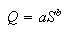where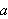and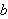are parameters that are fit using an power regression,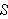is stage height in centimeters, and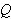is the discharge in L/s. Plot the data and the rating curve using linear axes for both the stage and discharge. Report the rating curve equation.

Q2) Plot the rating curve on log-log coordinates and show the stage - discharge records on the same plot. How well do these data match the curve when plotted in a log-log format?

## Stage-Discharge records

##### Some stage - discharge records for the channel at Martinelli Snowpatch, Niwot Ridge, Indian Peaks are included in the attached file

Q3) Plot the stage height vs. time for July 4 to 18 inc. (use a 10 minute sampling interval)

Q4) Use the rating curve to determine the discharge from July 4 to 18. Plot the discharge vs. time for July 4 to 18 inclusive. (use a 10-minute sampling interval)

Q5) Plot the air temperature for the same time period.

Q6) What date recorded peak flow during this period? Describe the variation in peak flow timing over the two weeks? How is it related to the temperature?

## Seasonal Discharge Hydrograph

Lets make a seasonal hydrograph using real data from the Martinelli catchment for 1994. You'll need to obtain this data from the Niwot Ridge LTER website:

select "Available Data" under Data and agree to the terms.

select Hydrology under "Metadata/data available for core topics" and search for data

select "Streamflow from Martinelli Basin, 1982-"

save as text or ascii file (should be the default) or copy and paste the desired date range into EXCEL.

Select the column with the text data and do "Text to Columns" under the Data tab. Choose comma delimited.

Q7) Make a seasonal hydrograph for 1994 using these data. Be sure to label axes and include units. Identify the rising limb and recession limb of the hydrograph.

Q8) On what day or days was peak discharge and what was the amount of peak discharge?

Q9) Estimate the recession coefficient as an average for the falling flows of the record. Use the data from period 7/14-8/6. The regression equation is of the form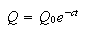whereis the discharge at the start of the regression time period andis the exponential regression coefficient. (*Hint: the regression coeffiecient has units!) Plot the regression curve along with the data for this time period.

To accomplish this, there is an example Excel spreadsheet. The example is for 1985 data, so you will need to replace the data (column B) with the appropriate time period. The model calculations (which use the recession curve equations) are in column D. By modifying the parameters Q0 and c (in cells D3 and D4), you can visually fit the model to the data. Cell E5 is the sum of squared error between the data on the model. The lower you can make this value the better your model fit is. The end result you are looking for are the values of Q0 and c that best match the model to the data. Don't forget to adjust the formula in E5 to include the whole dataset! *Hint: To get the best answer, try using the Solver in Excel to minimize the sum of the squared errors.

Q10) Provide a coherent guess as to why peak discharge has such a large and well-defined shape?

Q11) Why is the early summer discharge highly variable, compared to the discharge in late summer?